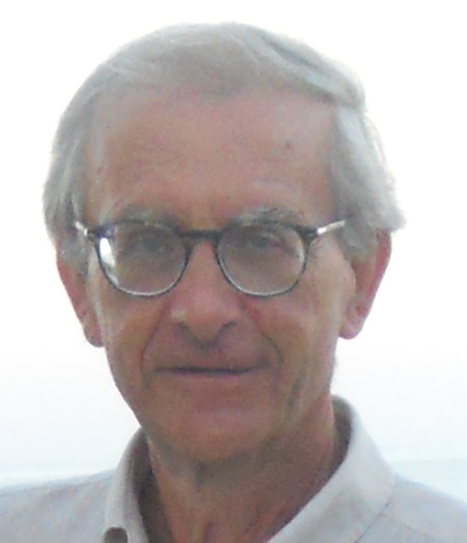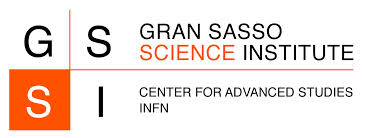Recent Results & Open Problems
in Mathematical Physicsa conference in honor of

Giuseppe Benfatto

February 13, 2015 at the Gran Sasso Science Institute at l'Aquila (Italy)

Venue: viale Francesco Crispi 7, 67100 L'Aquila (AQ) - GSSI Main Lecture Hall (directions)

Poster

Program

 10:30 Giovanni Gallavotti: "Modello gerarchico e effetto Kondo" (abstract) Abstract: (joint work with G. Benfatto and I. Jauslin) L'effetto Kondo consiste, fra l'altro, nella non divergenza per $T\to0$ della suscettività magnetica di una impurità con spin in interazione con un sistema di fermioni. Anche a 1 dimensione la teoria pertubativa, per interazione piccola, non dà risultati (ma divergenze): la teoria di Wilson, basata sul gruppo di rinormalizzazione, verrà applicata a un modello gerarchico. La relativa "funzione beta" è calcolata esattamente definendo un sistema dinamico con un punto fisso non banale e il flusso generato implica anche la finitezza della suscettività magnetica a $T=0$. 11:05 Benedetto Scoppola: "Sampling of Ising systems by reversible and irreversible probabilistic cellular automata" (abstract) Abstract: In this talk we discuss a class of probabilistic cellular automata, both reversible and irreversible, that are interesting in statistical mechanics. The reversible PCA has a stationary measure very close to the Gibbs measure of the Ising system. The irreversible case can be proven to have the same feature, being moreover an example of a dynamics with polynoomial mixing time in the low temperature regime. Joint works with Paolo Dai Prà, Carlo Lancia and Elisabetta Scoppola. 12:00 Alessandro Giuliani: "Height fluctuations in interacting dimers" (abstract) Abstract: (joint work with V. Mastropietro and F. Toninelli) Perfect matchings of $\mathbb Z^2$ (also known as non-interacting dimers on the square lattice) are an exactly solvable 2D statistical mechanics model. It is known that the associated height function behaves at large distances like a massless gaussian field, with the variance of height gradients growing logarithmically with the distance. As soon as dimers mutually interact, via e.g. a local energy function favoring the alignment among neighboring dimers, the model is not solvable anymore and the dimer-dimer correlation functions decay polynomially at infinity with a non-universal (interaction-dependent) critical exponent. We prove that, nevertheless, the height fluctuations remain gaussian even in the presence of interactions, in the sense that all their moments converge to the gaussian ones at large distances. The proof is based on a combination of multiscale methods with the path-independence properties of the height function. 12:35 Mario Pulvirenti: "On the Boltzmann equation for Fermions" (abstract) Abstract: I discuss the problem of deriving, in the weak-coupling limit, the Boltzmann equation for a system of Fermions. A stochastic toy model is also analyzed. 14:45 Guido Gentile: "Resonant tori of arbitrary codimension for quasi-periodically forced systems" (abstract) Abstract: Consider a system of rotators subject to a small quasi-periodic forcing which is analytic satisfies a time-reversibility property has a Bryuno frequency vector. Without imposing any non-degeneracy condition, we prove that there exists at least one quasi-periodic solution with the same frequency vector as the forcing. The result can be interpreted as a theorem of persistence of lower-dimensional tori of arbitrary codimension in degenerate cases. 15:20 Pierre Picco: "Phase Separation point for the one-dimensional Ising model with long range interactions" (abstract) Abstract: (joint work with M. Cassandro and T. Merola) We consider the phase separation problem for the one-dimensional ferromagnetic Ising model with long-range two-body interaction, $J (n) = n^{-2+\alpha}$, where $n$ denotes the distance of the two spins and $0\le\alpha<\alpha_+$, with $\alpha_+= (\log 3)/(\log 2)-1$. We prove that when $\alpha=0$ the localization of the phase separation fluctuates macroscopically with a non-uniform explicit limiting law, while when $0<\alpha<\alpha_+$ the macroscopic fluctuations disappear and mesoscopic ones appear with a gaussian behavior when conveniently scaled. 16:15 Vieri Mastropietro: "Anderson Localization in Many Body Interacting Quasi-Periodic Fermionic Chains" (abstract) Abstract: While Anderson localization is mathematically a rather well understood phenomenon when interaction between particles is neglected, our understanding of the interplay between localization and many body interaction, always present in physical systems, is quite poor. An extension of a technique introduced back in 1997 in Benfatto, Gentile, Mastropietro (JSP 1997) is quite useful for this problem. In particular, I consider a 1d many body fermionic system with an incommensurate external potential and a short range interaction. Assuming suitable choices of the chemical potential, strong potential, weak interaction and a Diophantine condition for the frequency, the zero temperature thermodynamical correlations are proved to be exponentially decaying for large distances, a property indicating persistence of localization in presence of interaction in the ground state. 16:50 Errico Presutti: "Phase transitions in highly anistotropic systems" (abstract)